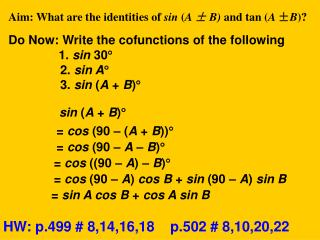# Aim: What are the identities of sin ( A ± B) and tan ( A ± B )? - PowerPoint PPT PresentationDownload PresentationAim: What are the identities of sin ( A ± B) and tan ( A ± B )?

Aim: What are the identities of sin ( A ± B) and tan ( A ± B )?Download Presentation## Aim: What are the identities of sin ( A ± B) and tan ( A ± B )?

- - - - - - - - - - - - - - - - - - - - - - - - - - - E N D - - - - - - - - - - - - - - - - - - - - - - - - - - -
##### Presentation Transcript

1. Aim: What are the identities of sin (A ± B) and tan (A ±B)? Do Now: Write the cofunctions of the following 1. sin 30 2. sin A 3. sin (A + B) sin (A + B) = cos (90 – (A + B)) = cos (90 – A – B) = cos ((90 – A) – B) = cos (90 – A) cos B + sin (90 – A) sin B = sin A cos B + cos A sin B HW: p.499 # 8,14,16,18 p.502 # 8,10,20,22

2. Let’s guess what sin (A – B) is equivalent to? sin (A – B) = sin A cos B – cos A sinB In additional to identities of the sum and difference of two angles of sine and cosine, let’s take a look at other identities. sin (-A) = cos (90 –(-A)) = cos (90 + A) = cos 90 cos A – sin 90 sin A = 0(cos A) – 1(sin A) = –sin A cos (-A) = sin (90 – (-A)) = sin (90 + A) = sin 90 cos A + cos 90 sin A = 1(cos A) + 0(sin A) = cos A

3. Example: Find the exact value of sin 105° Example: Find the exact value of sin 65cos 25 + cos 65 sin 25 sin(65 + 25) = sin 90° = 1

4. If A is a positive acute angle. B is a positive obtuse angle. Find the value of sin (A – B) sin (A – B) =sin A cos B – cos A sin B

5. Use the same way, we can prove that

6. Find the exact value of tan (A + B) and tan (A – B) A = 45 and B = 210 Find the exact value of tan 285 Find the exact value of tan 185